# AQA A Level Chemistry复习笔记3.6.2 Mass spectrometry

### Interpreting a Mass Spectrum

• Mass spectroscopy is an analytical technique used to identify unknown compounds
• The molecules in the small sample are bombarded with high energy electrons which can cause the molecule to lose an electron
• This results in the formation of a positively charged molecular ion with one unpaired electron
• One of the electrons in the pair has been removed by the beam of electrons• The molecular ion can further fragment to form new ions, molecules, and radicals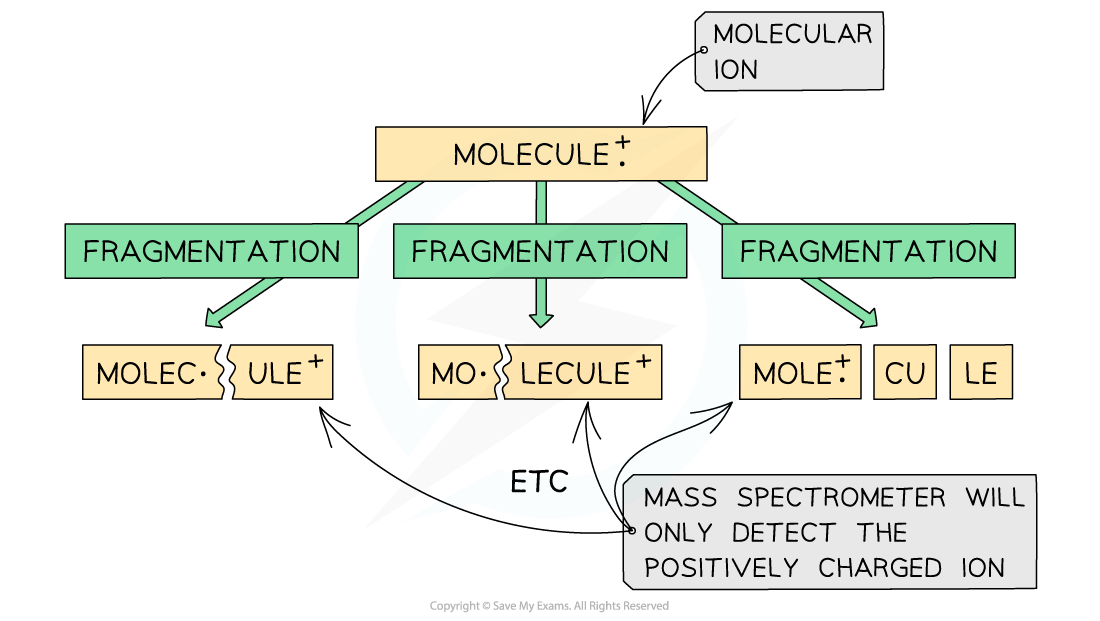Fragmentation of a molecule in mass spectroscopy

• These fragmented ions are accelerated by an electric field
• Based on their mass (m) to charge (z) ratio, the ion fragments are then separated by deflecting them into the detector
• Most ions will only gain a charge of 1+ and therefore a ion with mass 12 and charge 1+ will have an m/z value of 12
• It is, however, possible for a greater charge to occur. For example, an ion with mass 16 and charge 2+ will have a m/z value of 8

• The smaller and more positively charged fragment ions will be detected first as they will get deflected the most and are more attracted to the negative pole of the magnet
• Each fragment corresponds to a specific peak with a particular m/z value in the mass spectrum
• The base peak is the peak corresponding to the most abundant ion
• The m/z is sometimes referred to as the m/e ratio and it is almost always 1:1

#### Isotopes

• Isotopes are different atoms of the same element that contain the same number of protons and electrons but a different number of neutrons.
• These are atoms of the same elements but with different mass number
• For example, Cl-35 and Cl-37 are isotopes as they are both atoms of the same element (chlorine, Cl) but have a different mass number (35 and 37 respectively)

• Mass spectroscopy can be used to find the relative abundance of the isotopes experimentally
• The relative abundance of an isotope is the proportion of one particular isotope in a mixture of isotopes found in nature
• For example, the relative abundance of Cl-35 and Cl-37 is 75% and 25% respectively
• This means that in nature, 75% of the chlorine atoms is the Cl-35 isotope and 25% is the Cl-37 isotope

• The heights of the peaks in mass spectroscopy show the proportion of each isotope presentThe peak heights show the relative abundance of the boron isotopes: boron-10 has a relative abundance of 19.9% and boron-11 has a relative abundance of 80.1%

#### Worked Example

Calculating m/z ratio

In a sample of iron, the ions 54Fe2+ and 56Fe3+ are detected. Calculate their m/z ratio and determine which ion is deflected more inside the spectrometer.• 56Fe3+ has a smaller m/z ratio and will therefore be deflected more.
• It also has the largest positive charge and will be more attracted to the negative pole of the magnet within the mass spectrometer.

#### Exam Tip

A small m/z value corresponds to fragments that are either small or have a high positive charge or a combination of both.

### Deducing Molecular Formula

• Each peak in the mass spectrum corresponds to a certain fragment with a particular m/z value
• The peak with the highest m/z value is the molecular ion (M+) peak which gives information about the molecular mass of the compound
• The molecular ion is the entire molecule that has lost one electron when bombarded with a beam of electrons• The [M+1] peak is a smaller peak which is due to the natural abundance of the isotope carbon-13
• The amount of naturally occurring C-13 is a little over 1%, so the [M+1] peak is very small
• The height of the [M+1] peak for a particular ion depends on how many carbon atoms are present in that molecule; the more carbon atoms, the larger the [M+1] peak is
• For example, the height of the [M+1] peak for an hexane (containing six carbon atoms) ion will be greater than the height of the [M+1] peak of an ethane (containing two carbon atoms) ion

#### Worked Example

Analysing mass spectra

Determine whether the following mass spectrum corresponds to but-1-ene or pent-1-ene: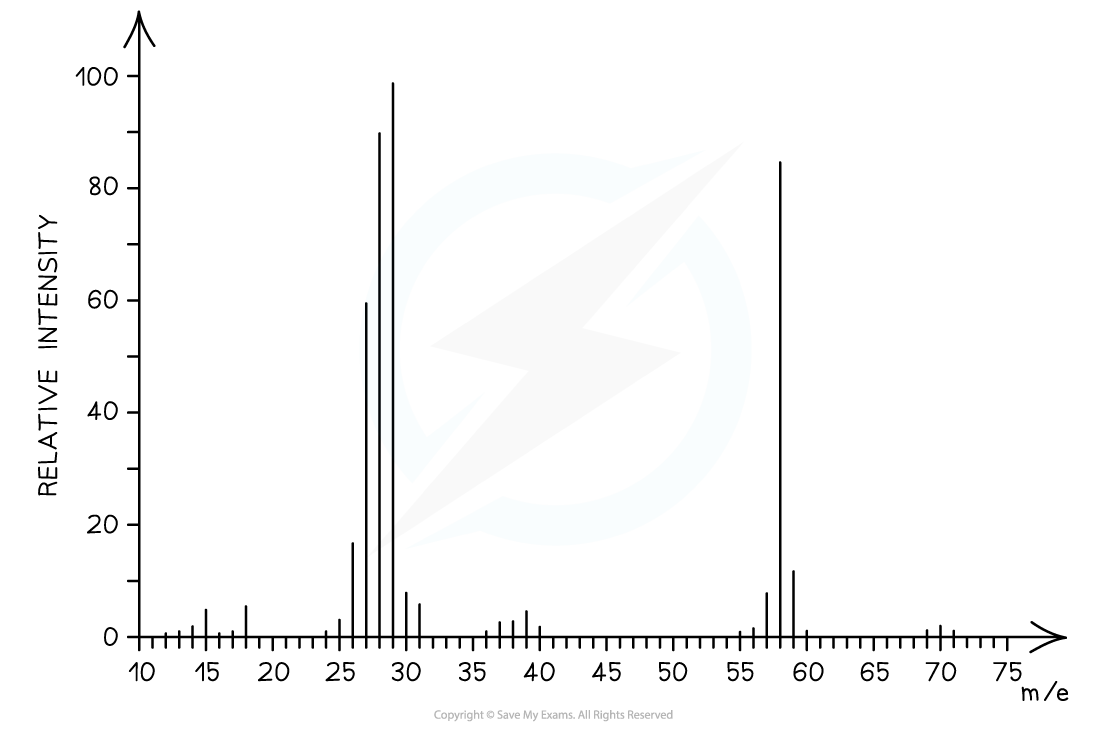• The mass spectrum corresponds to pent-1-ene as the molecular ion peak is at m/z = 70
• The small peak at m/z = 71 is a C-13 peak, which does not count as the molecular ion peak
• But-1-ene arises from the C4H8+ ion which has a molecular mass of 56
• Pent-1-ene arises from the C5H10+ ion which has a molecular mass of 70

### Fragmentation

• The molecular ion peak can be used to identify the molecular mass of a compound
• However, different compounds may have the same molecular mass
• To further determine the structure of the unknown compound, fragmentation is used
• Fragments may appear due to the formation of characteristic fragments or the loss of small molecules
• For example, a peak at 29 is due to the characteristic fragment C2H5+­­
• Loss of small molecules give rise to peaks at 18 (H2O), 28 (CO), and 44 (CO2)

#### Alkanes

• Simple alkanes are fragmented in mass spectroscopy by breaking the C-C bonds
• M/e values of some of the common alkane fragments are given in the table below

m/e Values of Fragments Table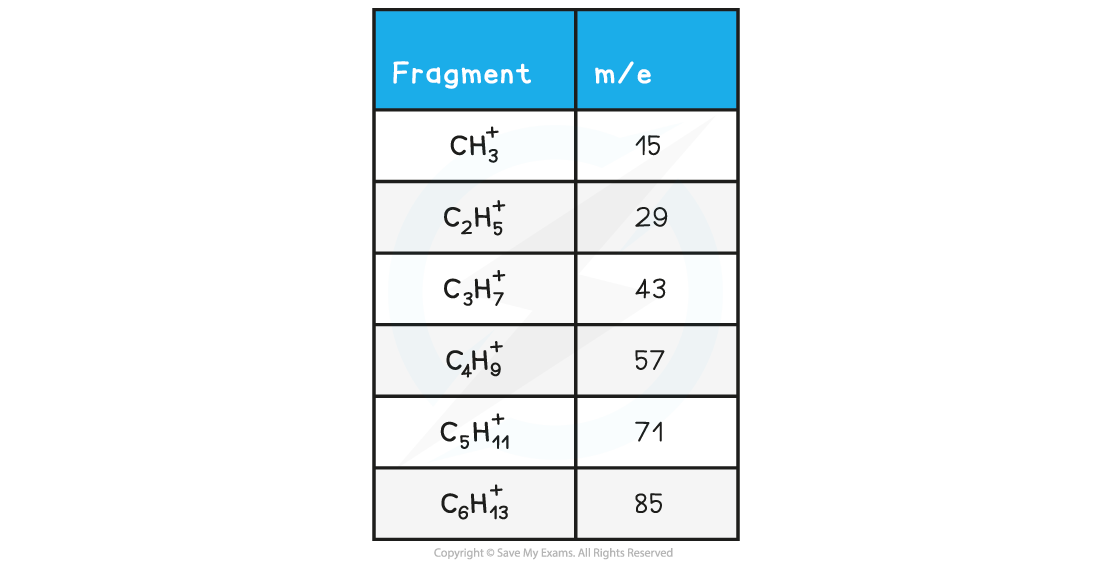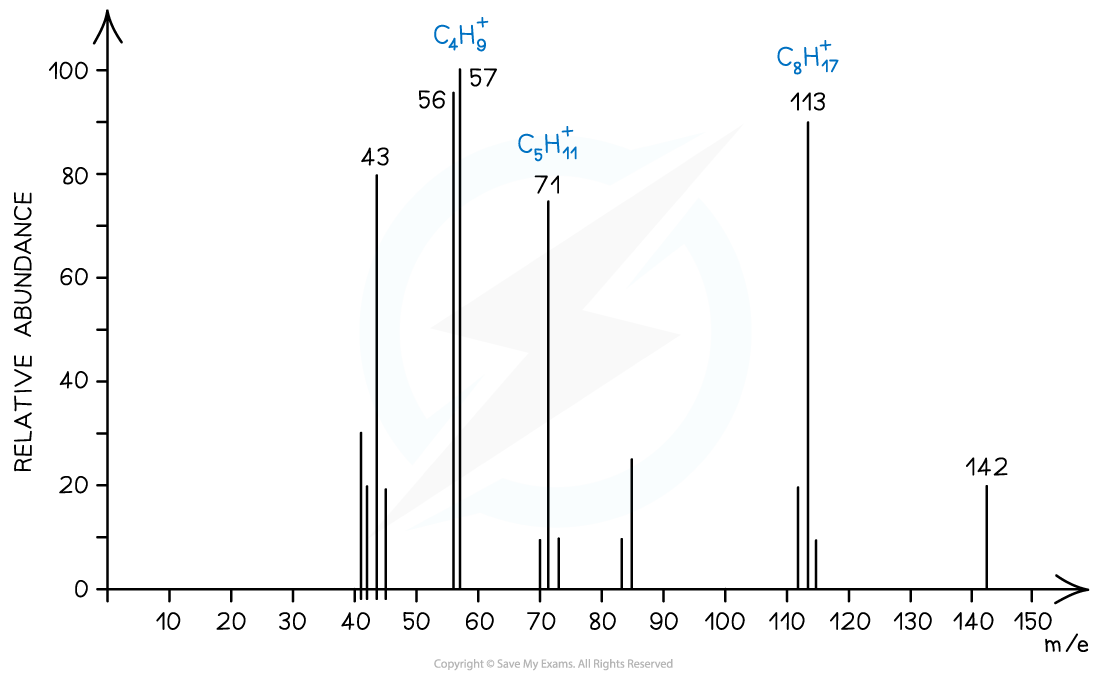Mass spectrum showing the fragmentation of C10H22

#### Halogenoalkanes

• Halogenoalkanes often have multiple peaks around the molecular ion peak
• This is caused by the fact that there are different isotopes of the halogens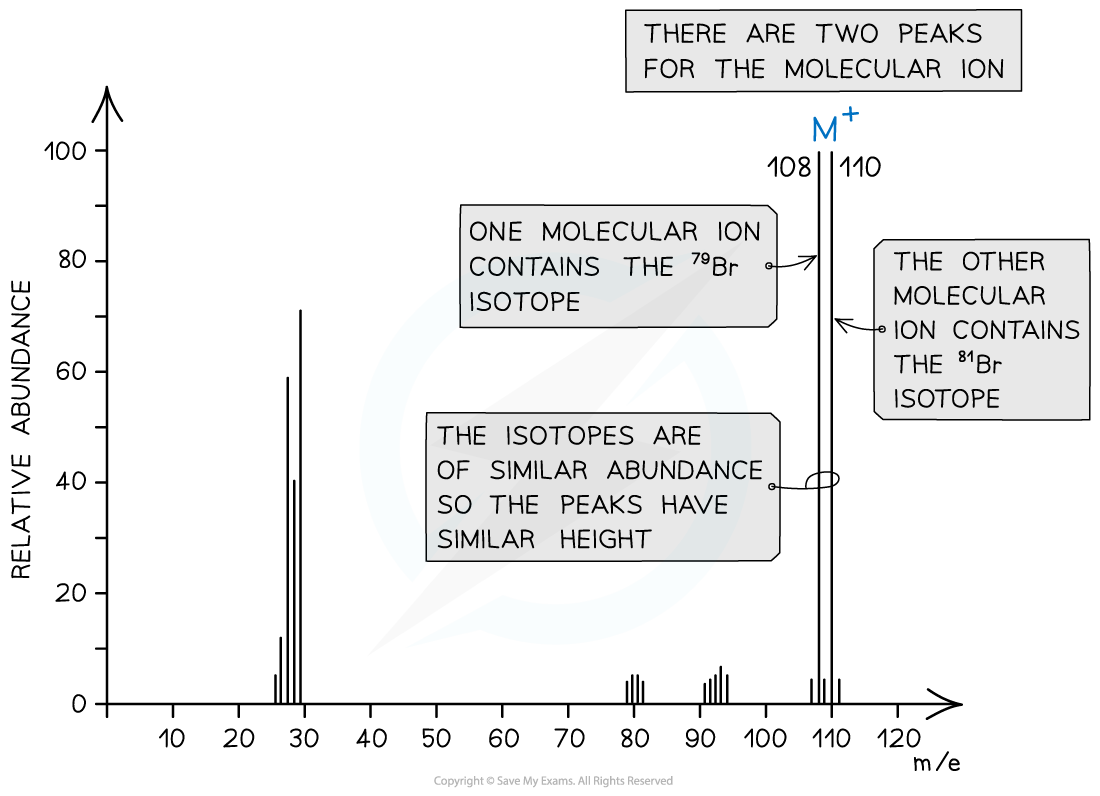Mass spectrum showing different isotopes of bromine in the molecular ion

#### Alcohols

• Alcohols often tend to lose a water molecule giving rise to a peak at 18 below the molecular ion
• Another common peak is found at m/e value 31 which corresponds to the CH2OH+­­ fragment
• For example, the mass spectrum of propan-1-ol shows that the compound has fragmented in four different ways:
• Loss of H• to form a C3H7O+ fragment with m/e = 59
• Loss of a water molecule to form a C3H6+ fragment with m/e = 42
• Loss of a •C2H5 to form a CH2OH+ fragment with m/e = 31
• And the loss of •CH2OH to form a C2H5+ fragment with m/e = 29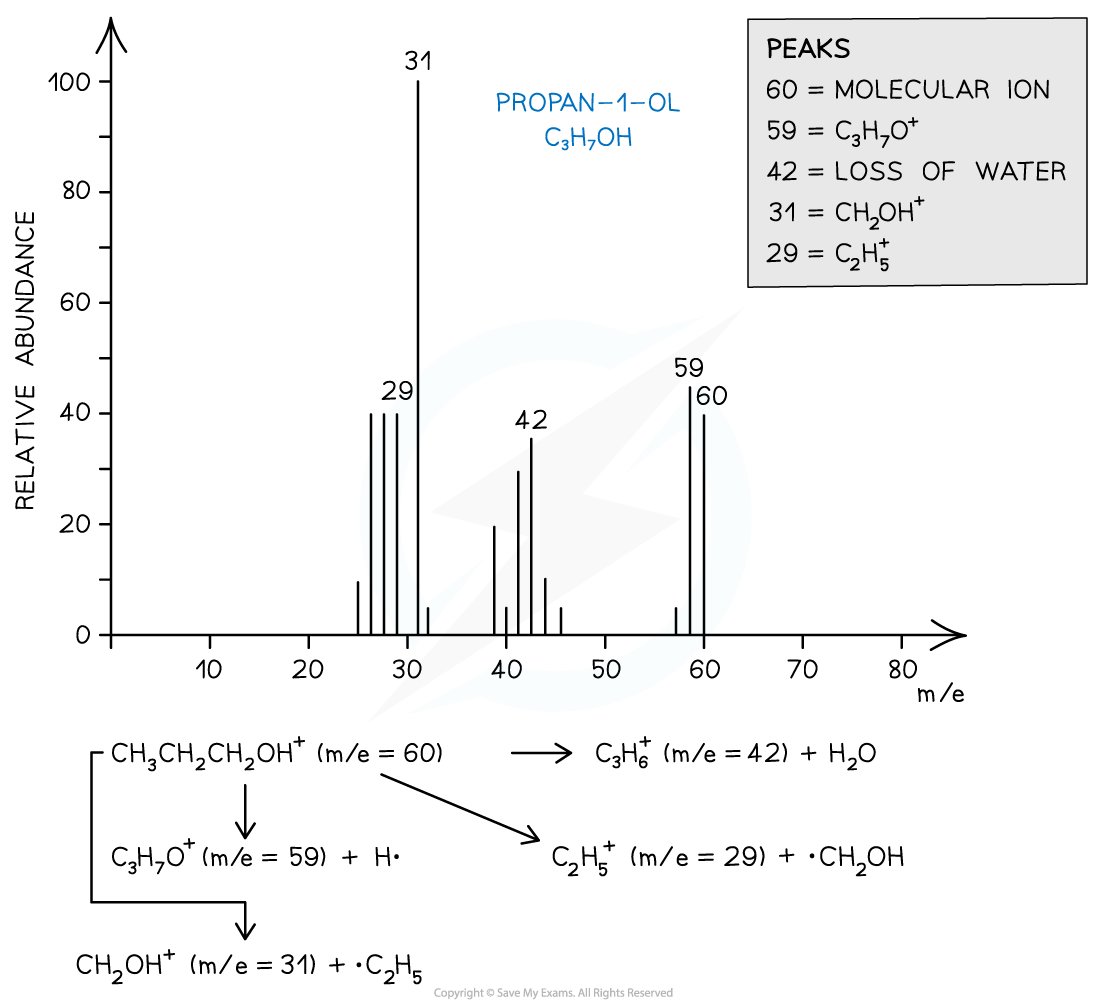Mass spectrum showing the fragmentation patterns in propan-1-ol (alcohol)

#### Worked Example

Ion fragmentation

Which of the following statements about the mass spectrum of CH3Br is correct?

A. There is one peak for the molecular ion with an m/e value of 44

B. There is one peak for the molecular ion with an m/e value of 95

C. The last two peaks have abundances in the ratio 3:1 and occur at m/e values of 94 and 96

D. The last two peaks are of equal size and occur at m/e values of 94 and 96

The correct answer is option D

• Bromomethane (CH3Br) can produce 3 peaks
• CH381Br → [CH381Br]+ + e− at m/e 96
• CH379Br → [CH379Br]+ + e− at m/e 94
• CH3Br → [CH3]+ + •Br at m/e 15

• The last two peaks (which correspond to the molecular ion peak) therefore are equal in size and occur at m/e values of 94 and 96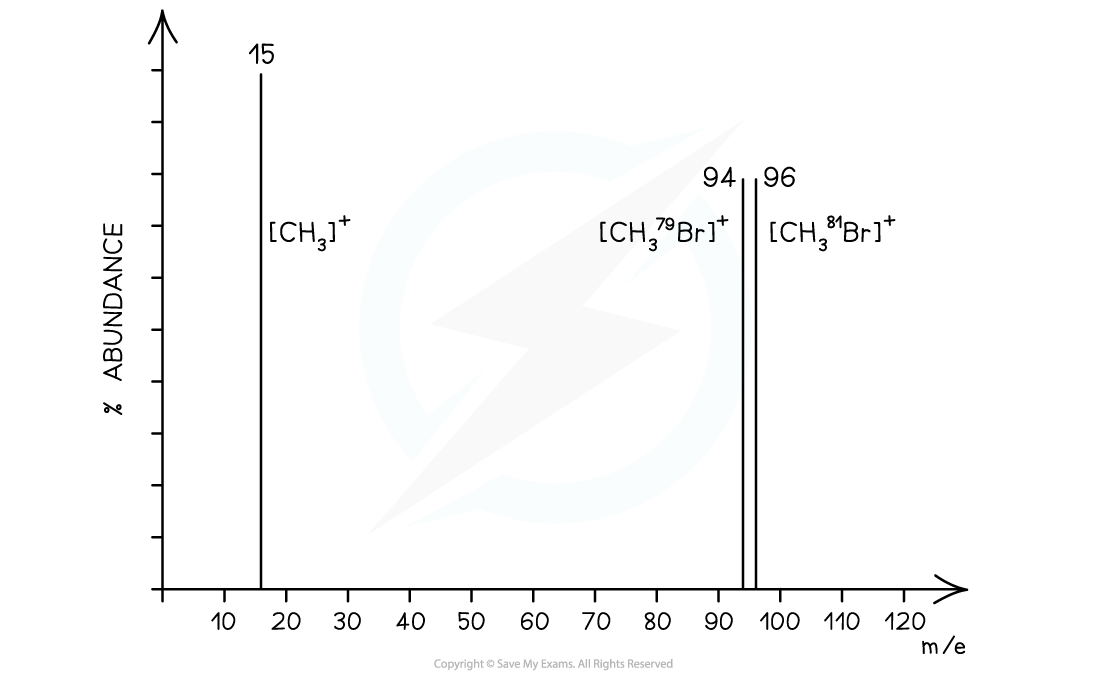#### Worked Example

Alcohol fragmentation

Which alcohol is not likely to have a fragment ion at m/e at 43 in its mass spectrum?

A. (CH3)2CHCH2OH

B. CH3CH(OH)CH2CH2CH3

C. CH3CH2CH2CH2OH

D. CH3CH2CH(OH)CH3

The correct answer is option D

• Because a line at m/e = 43 corresponds to an ion with a mass of 43 for example:
• [CH3CH2CH2]+
• [(CH3)2CH]+

• 2-butanol is not likely to have a fragment at m/e = 43 as it does not have either of these fragments in its structure.